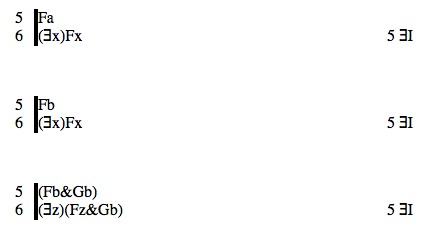# Tutorial 20: Existential Introduction

Logical System

2013

Bergmann The Logic Book Section 10.1.

### The Tutorial

There is a rule for adding a Existential Quantifier, Existential Introduction (also commonly known as 'Existential Generalization'). This permits the step illustrated by the following proof fragments.To understand what this rule permits, it helps to think about it as though you were going backwards from line 6 to line 5. Take the scope of line 6, which is Fx, Fx, and (Fz&Gb) respectively, then substitute a constant for free occurrences of the variable of quantification-- you should be able to get line 5, and indeed you can.

#### The Rule of Existential Introduction ∃I

If a derivation contains a line of the form

n. <formula>[<constant>/<variable>] <any justification>

then a line of the form

(∃<variable>)<formula> 'n ∃I'

may be added to the derivation.

Notice that, in a sense, you are generalizing on occurrences of the constant in the first formula. Now, you do not have to generalize on all of them; you can generalize on 0, 1, or many, depending on what is there and what you want to do.

## Exercise to accompany Predicate Tutorial 10

### Exercise 1 (of 1)

Derive the following

a) Fb∴(∃x)Fx
b) Fa∴(∃y)Fy
c) Fa∴(∃x)Fa
d) Fa,Ga∴(∃x)(Fx&Gx)
e) Fa,Ga∴(∃x)(Fx&Ga)
f) Fa,Ga∴(∃x)(Fa&Gx)
g) Fa,Ga∴(∃x)(Fx)&Ga

If you decide to use the web application for the exercises you can launch it from here Deriver[Bergmann] — username 'logic' password 'logic'. Then either copy and paste the above formulas into the Journal or use the Deriver File Menu to Open Web Page with this address https://softoption.us/test/easyDeriver/CombinedExercisesEasyDBergmann.html .

Preferences

You may need to set some Preferences for this.

• you can check that the parser is set to bergmann.